# Relations and Functions

## 6 . Type Of Functions:

### One to One Function or Injective :

Let f: A -> B, a function from a set A to a set B, f is called a one-to-one function or injection, if, and only if, for all elements a1 and a2 in A,
if f(a1) = f(a2), then a1 = a2

Equivalently,

if a1 ≠ a1, then f(a1) ≠ f(a2).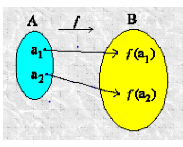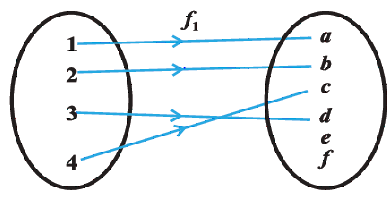A function is not one on one if this condition is met, then it is called many one function
f(a1) = f(a2), then a1 ≠ a2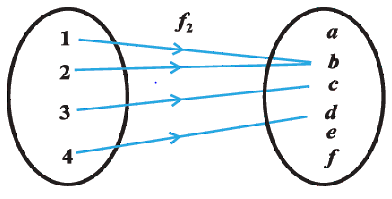#### How to Prove a Function for One to One:

1) We have to prove that
if f(a1) = f(a2), then a1 = a2

2) Let us take example as
f : R-> R
f(x) =3x-2 for x ∈ R
3) f(a1) =3a1-2
f(a2)=3a2-2

Now f(a1) = f(a2),

3a1-2=3a2-2
or a1 = a2
So our first point is proved,so this is one to one function

#### How to prove a function for Many one 's ( Not one to one)

1) We have to prove that

if f(a1) = f(a2), then a1 ≠ a2
2) We can prove this by giving counter example
f : R-> R
f(x) =x2 for x ∈ R
Now for x=2 f(2)=4
for x=-2 ,f(-2)=4
Now f(2)=f(-2) Hence Many one

### Onto (Surjective ) Function:

A function f: A-> B is said to be onto(surjective) if every element of B is the image of some element of A under f, i,e
for every y ∈ B, there exists a element x in A where f(x)=y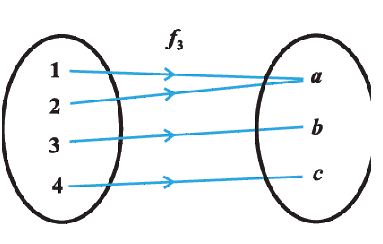#### How to Prove a Function for Onto (Surjectivity):

1) for every y ∈ B, there exists a element x in A where f(x)=y
2) Let us take example as
f : R-> R
f(x) =3x-2 for x ∈ R
3) Let y ∈ R, then we first need to prove that we can find x ∈ R such that f(x)=y
If such real number x exists then y=3x-2
or x=(y+2)/3
Now sum and division of real number is a real number,So x ∈ R
it follows
f((y+2)/3 )= 3(y+2)/3 -2=y

hence f is onto function

4) if we have to prove that function is not onto,then easiast way would be to take counter examples

### Bijective (One on One and onto) Function

A function is said to be Bijective if it is both one on one and onto function.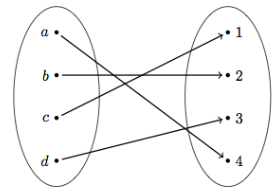#### How to Prove a Function for Bijectivity

To prove a function is bijective, you need to prove that it is injective and also surjective.
"Injective" means no two elements in the domain of the function gets mapped to the same image.The method has been already described above.
"Surjective" means that any element in the range of the function is hit by the function.The method has been already described above.

Go back to Class 12 Main Page using below links